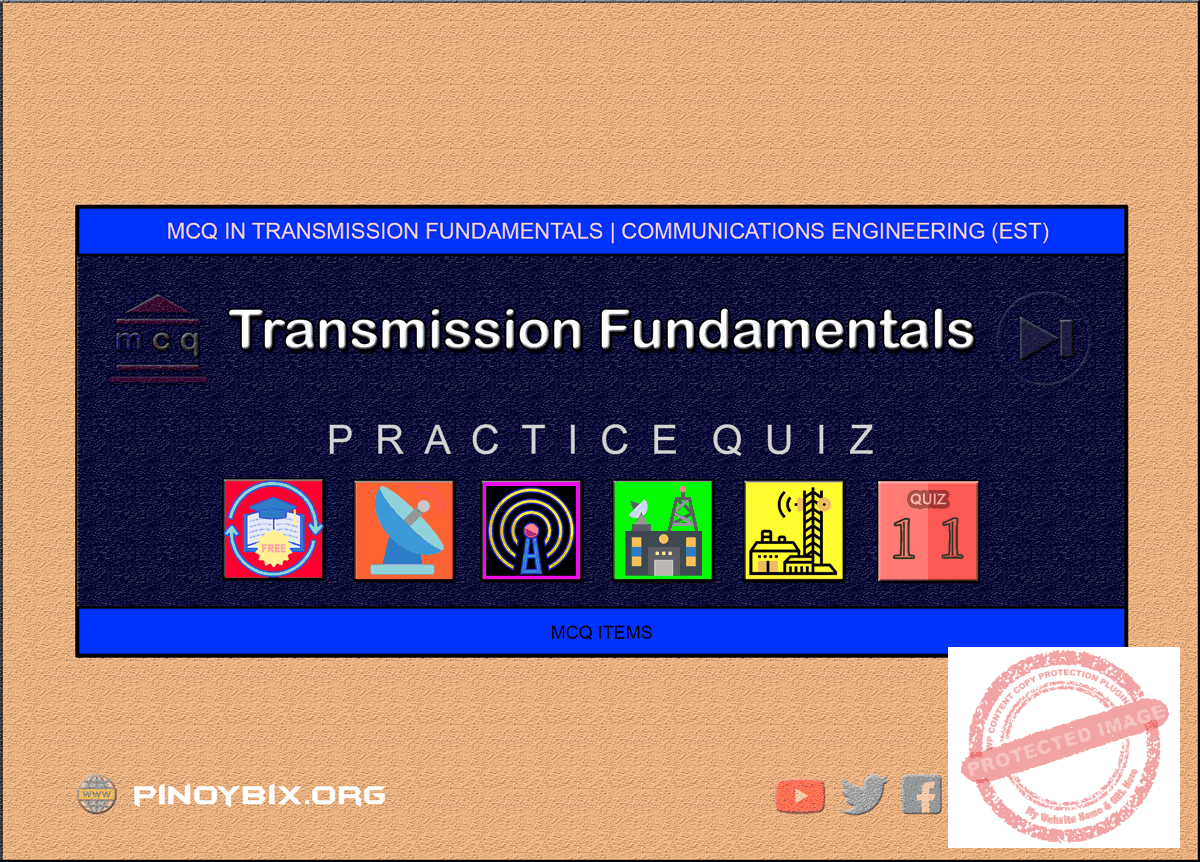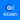# MCQ in Transmission Fundamentals Part 11 | ECE Board Exam

(Last Updated On: May 6, 2020)This is the Multiples Choice Questions Part 11 of the Series in Transmission Fundamentals as one of the Communications Engineering topic. In Preparation for the ECE Board Exam make sure to expose yourself and familiarize in each and every questions compiled here taken from various sources including but not limited to past Board Examination Questions in Electronic System and Technologies, Communications Books, Journals and other Communications References.

#### MCQ Topic Outline included in ECE Board Exam Syllabi

• MCQ in Transmission System
• MCQ in Transmission Medium
• MCQ in Velocity and Line Wavelength
• MCQ in Characteristics Impedance
• MCQ in Primary Line Constants and Propagation Constants
• MCQ in Standing Waves
• MCQ in Voltage Standing Wave Ratio
• MCQ in Telephone Lines and Cables
• MCQ in Wave Guides
• MCQ in Balanced and Unbalanced Lines
• MCQ in Twisted pair wire, Coaxial Cable
• MCQ in Decibel
• MCQ in Signal and Noise Fundamentals

#### Continue Practice Exam Test Questions Part 11 of the Series

501. A (75 – j50) Ω load is connected to a coaxial transmission line of Zo = 75 Ω at 10 GHz. The best method of matching consists in connecting

a. A short circuited stub

b. An inductance at the load

c. A capacitance at some specific distance from the load

d. A short circuited stub at some specific distance from the load

Solution:

502. The velocity factor of a transmission line

a. Depends on the dielectric constant of the material used

b. Increases the velocity along the transmission line

c. Is governed by the skin effect

d. Is higher for a solid dielectric than for air

Solution:

503. What is the characteristic impedance of a single wire with diameter d = 0.25 mm placed at the center between grounded parallel planes separated by 1 mm apart. The wire is held by a material with a velocity factor of 0.75

a. 85 ohms

b. 63 ohms

c. 50 ohms

d. 75 ohms

Solution:

504. Coaxial lines are used on those system operating ________.

a. Above 10 GHz

b. At 300 MHz

c. Above 10 KHz

d. Below 2 GHz

Solution:

505. Referred to the dielectric constant of a transmission line material

a. Inductance and capacitance

b. Velocity factor

c. Characteristic impedance

d. Propagation velocity

Solution:

506. The main advantage of the two-hole directional coupler is

a. Low directional coupling

b. Poor directivity

c. High SWR

d. Narrow bandwidth

Solution:

507. To couple a coaxial line to a parallel-wire line, it is best to use a

a. Slotted line

b. Balun

c. Directional coupler

d. Quarter-wave transformer

Solution:

508. What is the meaning of the term velocity factor of a transmission line?

a. The index of shielding for coaxial cable

b. The velocity of the wave on the transmission line multiplied by the velocity of light in a vacuum

c. The ratio of the characteristic impedance of the line to the terminating impedance

d. The velocity of the wave on the transmission line divided by the velocity of light in a vacuum

Solution:

509. A transmission line consisting of two conductors that have equal resistance per unit length.

a. Unbalance line

b. Open-wire line

c. Balanced line

d. Coaxial line

Solution:

510. Which if the following is not a common transmission line impedance?

a. 50 Ω

b. 75 Ω

c. 120 Ω

d. 300 Ω

Solution:

511. For a maximum absorption of power at the antenna, the relationship between the characteristic impedance of the line Zo and the load impedance Zl should be

a. Zo = ZL

b. Zo > ZL

Solution:

[/toggle]

512. Which of the following determines the characteristics of a transmission line?

a. Inductance

b. Capacitance

c. Physical dimensions

d. Length

Solution:

513. In the study of transmission cable, twin lead is also referred to as a ______ .

a. Twisted pair

b. Double cable

c. Ribbon cable

d. Open pair

Solution:

514. A 50 Ω coax is connected to a 73 Ω the SWR is

a. 0.685

b. 1

c. 1.46

d. 2.92

Solution:

515. A ratio expressing the percentage of incident voltage reflected on a transmission line is known as the

a. Velocity factor

b. Standing-wave ratio

c. Reflection coefficient

d. Line efficient

Solution:

516. Three feet is one wavelength at a frequency of

a. 100 MHz

b. 164 MHz

c. 300 MHz

d. 328 MHz

Solution:

517. An open quarter-wave line at the operating frequency acts like a(n)

a. Series resonant circuit

b. Parallel resonant circuit

c. Capacitor

d. Inductor

Solution:

518. A shorted half-wave line at the operating frequency acts like a(n)

a. Capacitor

b. Inductor

c. Series resonant circuit

d. Parallel resonant circuit

Solution:

519. At UHF and microwave frequencies, transmission lines are commonly used as

a. Antenna

b. Coupler

c. Resonant circuit

d. Transformer

Solution:

520. The transmission medium subjects the transmitted signal to be

a. Modulated

b. Amplified

c. Attenuated

d. Boosted

Solution:

521. Transmission line is a two-wire cable that connects the transmitter to the antenna or the antenna to the receiver. The purpose of the transmission line is to

a. Amplify the RF signal

b. Modulate the signal

c. Carry the RF energy for the desired distance

d. All of the above

Solution:

522. These conductors are uniformly spaced by insulators. They have low losses at low medium frequencies and are economical to construct and maintained

a. Coaxial cables

b. Open-wire transmission lines

c. Optical cables

d. Waveguides

Solution:

523. To prevent radiation losses and interference from external sources, the inner conductor of this transmission line is completely enclosed with a metal sheath or braid

a. Coaxial cable

b. Open-wire transmission lines

c. Optical cables

d. Waveguides

Solution:

524. The term “balanced line” means that

a. Both conductors carry currents that flow in opposite directions

b. Conductors present equal capacitance to ground

c. One conductor is connected to ground

d. Both A and B

Solution:

525. The characteristic impedance of a transmission line is determined by:

a. Its operating frequency

b. Its length

c. Its physical size and arrangement

d. The signal applied at the input terminal

Solution:

526. The disturbance parameters of the transmission line are: the series resistance; the series inductance; the shunt capacitance and the shunt conductance. What parameter was determined due to dielectric losses?

a. Series resistance

b. Series inductance

c. Shunt capacitance

d. Shunt conductance

Solution:

527. Shunt capacitance was determined due to:

a. Conductors were closed to each other

b. The length and diameter of the conductors

c. Dielectric loses

Solution:

528. Series resistances was determined due to:

a. Conductors were closed to each other

b. The length and diameter of the conductors

c. Dielectric losses

Solution:

529. At radio frequencies was determined due to:

a. Characteristic

b. Inductive reactance is much larger than the shunt conductance

c. Capacitive reactance is much larger than the shunt conductance

d. Both b and c

Solution:

530. Determine the characteristic impedance of a transmission line which has a capacitance of 30 pF/m and an inductance of 75 nH/m

a. 5 Ω

b. 50 Ω

c. 500 Ω

d. 5 K Ω

Solution:

531. If a charge in the dielectric material decreases the capacitance, the characteristic impedance is

a. Kept constant

b. Decreased

c. Increased

d. Halved

Solution:

#### Questions and Answers in Transmission Fundamentals Series

Following is the list of multiple choice questions in this brand new series:

MCQ in Transmission Fundamentals
PART 1: MCQ from Number 1 – 50                        Answer key: PART 1
PART 2: MCQ from Number 51 – 100                   Answer key: PART 2
PART 3: MCQ from Number 101 – 150                 Answer key: PART 3
PART 4: MCQ from Number 151 – 200                 Answer key: PART 4
PART 5: MCQ from Number 201 – 250                 Answer key: PART 5
PART 6: MCQ from Number 251 – 300                 Answer key: PART 6
PART 7: MCQ from Number 301 – 350                 Answer key: PART 7
PART 8: MCQ from Number 351 – 400                 Answer key: PART 8
PART 9: MCQ from Number 401 – 450                 Answer key: PART 9
PART 10: MCQ from Number 451 – 500                Answer key: PART 10

### Complete List of MCQ in Communications Engineering per topic

Help Me Makes a Difference!

 P inoyBIX educates thousands of reviewers/students a day in preparation for their board examinations. Also provides professionals with materials for their lectures and practice exams. Help me go forward with the same spirit. “Will you make a small gift today via GCASH?”+63 966 459 6474 Option 1 : \$1 USD Option 2 : \$3 USD Option 3 : \$5 USD Option 4 : \$10 USD Option 5 : \$25 USD Option 6 : \$50 USD Option 7 : \$100 USD Option 8 : Other Amount© 2014 PinoyBIX Engineering. © 2019 All Rights Reserved | How to Donate? |